﻿ 2-dimensional analysis of a cylindrical mixer - XSim

# 2-dimensional analysis of a cylindrical mixer

Update: June 1, 2017
OpenFOAM 4.x

## Case directory

\$FOAM_TUTORIALS/incompressible/simpleFoam/mixerVessel2D

## Summary

We analyze a mixer as shown in the figure below using MRF function. The region "rotor" rotates at 104.72 rad/s with the Z-axis as the axis of rotation, and the calculation is performed as a two-dimensional problem with a single mesh in the Z direction.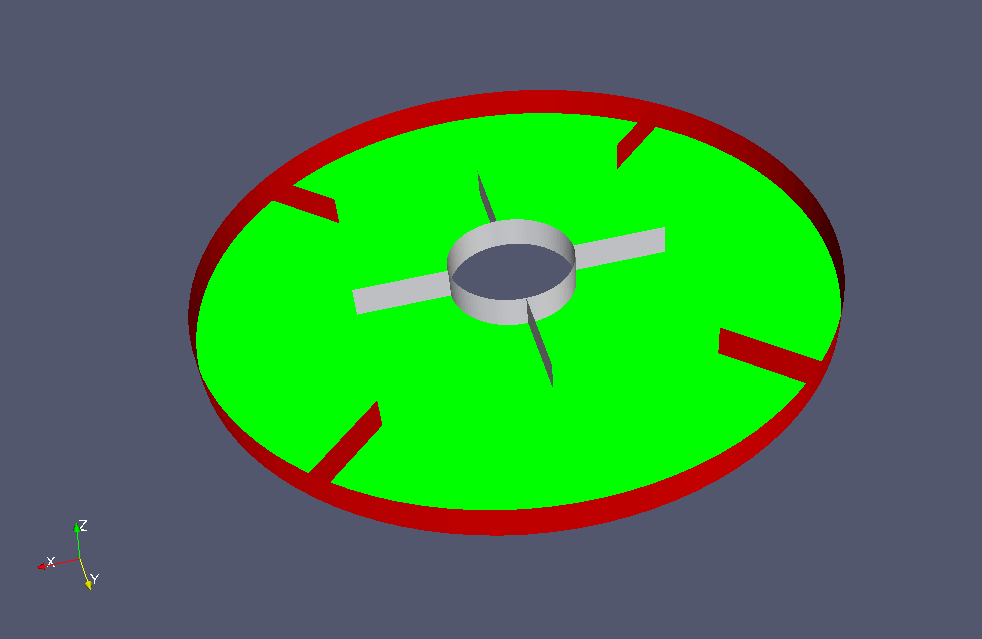Model geometry

The rotation axis and the rotation speed are defined in the file constant/MRFProperties as follows.

```MRF1
{
cellZone    rotor;
active      yes;

nonRotatingPatches ();

origin    (0 0 0);
axis      (0 0 1);
omega     104.72;
}
```

The rotation area is defined as the cell zone "rotor" as shown in the figure below (white part).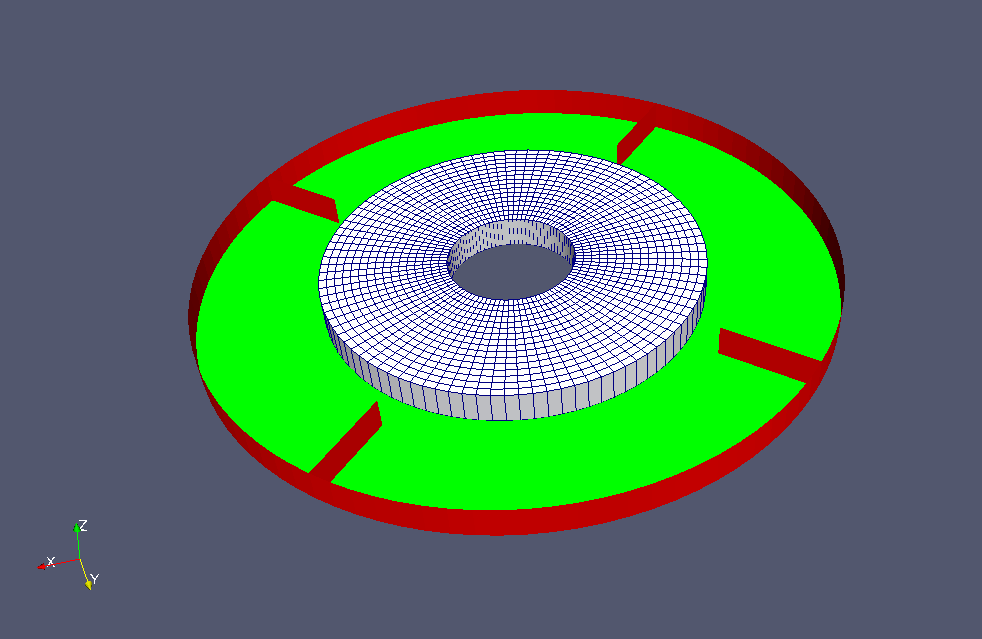Cell zone "rotor"

The standard k-ε model is used for the turbulence model.

The meshes are as follows, and the number of mesh is 3072.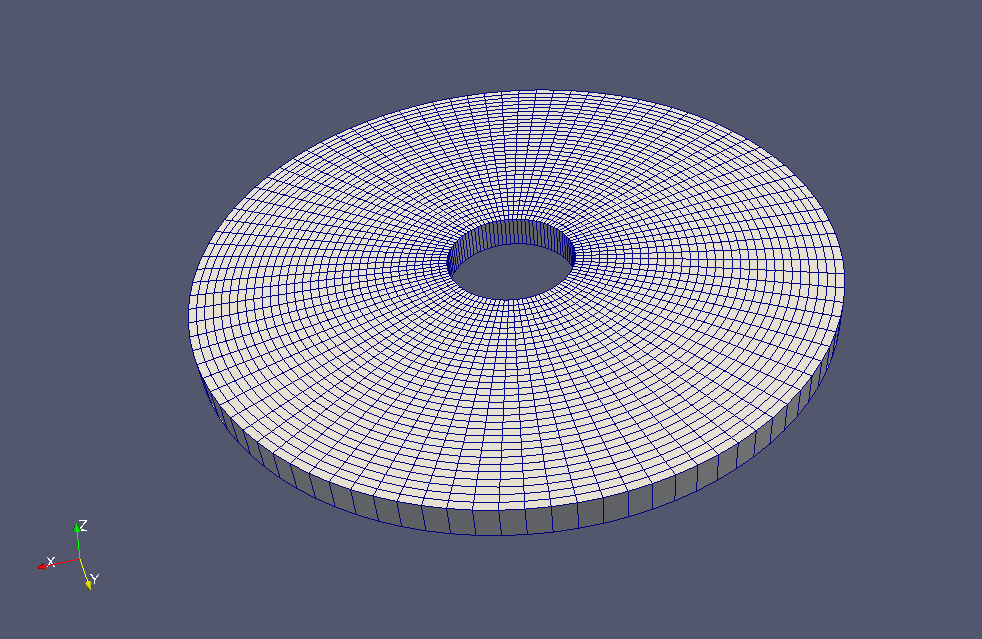Meshes

The calculation result is as follows.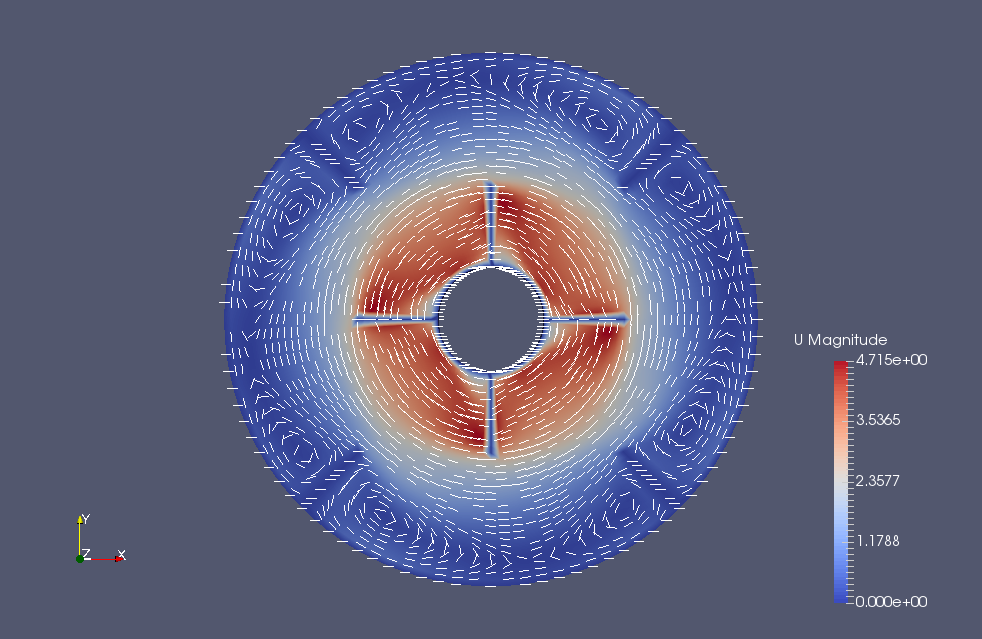Flow velocity (U)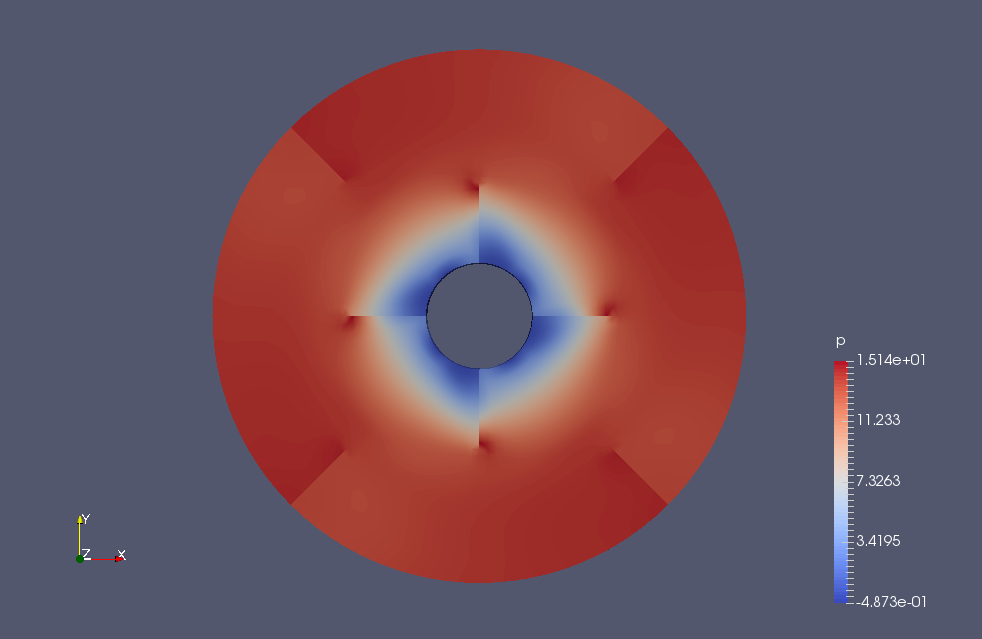Pressure (p)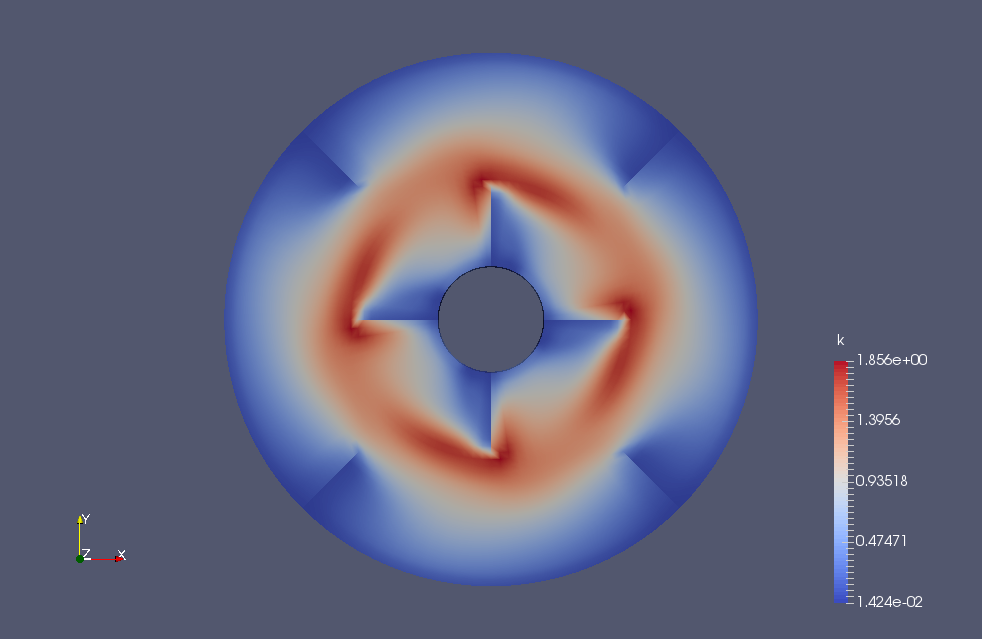Turbulent energy (k)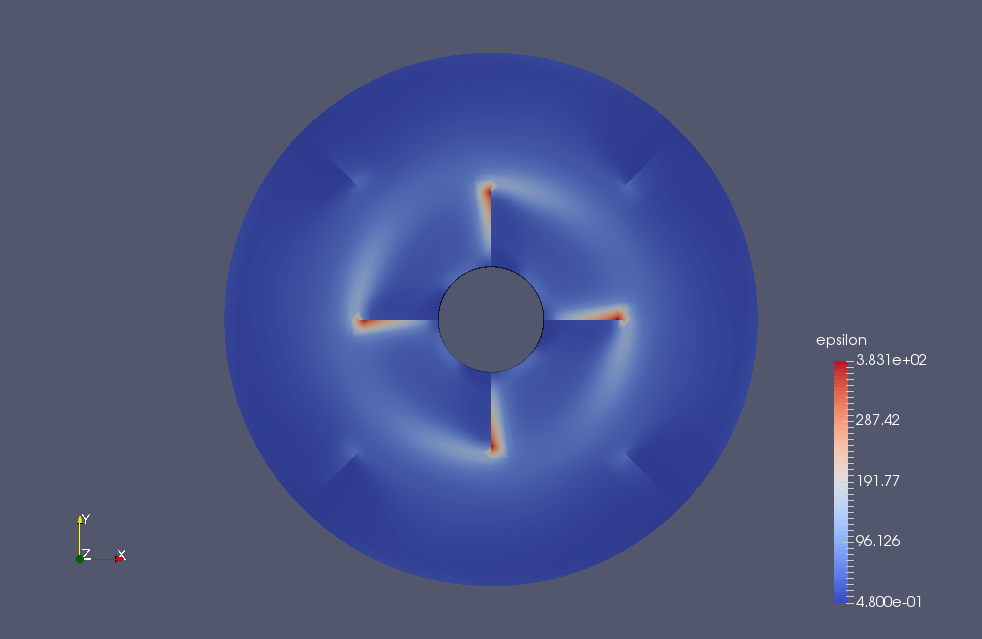Turbulent dissipation rate (epsilon)

## Commands

cp -r \$FOAM_TUTORIALS/incompressible/simpleFoam/mixerVessel2D mixerVessel2D
cd mixerVessel2D

m4 < system/blockMeshDict.m4 > system/blockMeshDict
blockMesh
simpleFoam

paraFoam

The macro language processor m4 is used to create the mesh. We run m4 with the prepared file "system/blockMeshDict.m4", and the output is saved in "system/blockMeshDict". After that, we run blockMesh to create the mesh for the calculation.

## Calculation time

5.41 seconds *Single, Inter(R) Core(TM) i7-2600 CPU @ 3.40GHz 3.40GHz